Home | | Engineering Mathematics I | Three Dimensional Analytical Geometry

# Three Dimensional Analytical Geometry

Formulae, Cone, Right-Circular Cone, Cylinder, Right-Circular Cylinder, The Sphere.

THREE DIMENSIONAL ANALYTICAL GEOMETRY

Formulae

Cone

Right-Circular Cone

Cylinder

Right-Circular Cylinder

The Sphere

FORMULAE:

1. The equation of the straight line through the point p(x1,y2,z1) and having direction cosines2. The equation of the straight line through the point B(x2,y2,z2) and having direction ratios3. The equation of the straight line passing through the points A(x1,y1,z1) and B(x2,y2,z2) is4. Angle between the straight lines:CONE

DEFINITION: A cone is defined as a surface generated by a straight line which passes through a fixed point and satisfies one or more conditioni.e.ie, it may intersect a fixed curve.

Note:

1. The fixed point is said to be the vertex of the cone

2. The fixed curve is said to be the guiding curve of the cone

3. The straight line in any position is called the generator of the cone.

FORMULA:

The equation of the cone with vertex (x1,y2,z1) and whose generators intersect the guiding curve1. Find the equation of the cone with vertex at (1,1,1) and which passes through the curve given byRIGHT-CIRCULAR CONE

DEFINITION: A right circular cone is a surface generated by a straight line which passes through a fixed point and makes a constant angle with a fixed line through the fixed point.  The equation of right circular cone vertex is (x1,y1,z1) ,the semi vertical angle a and axis the line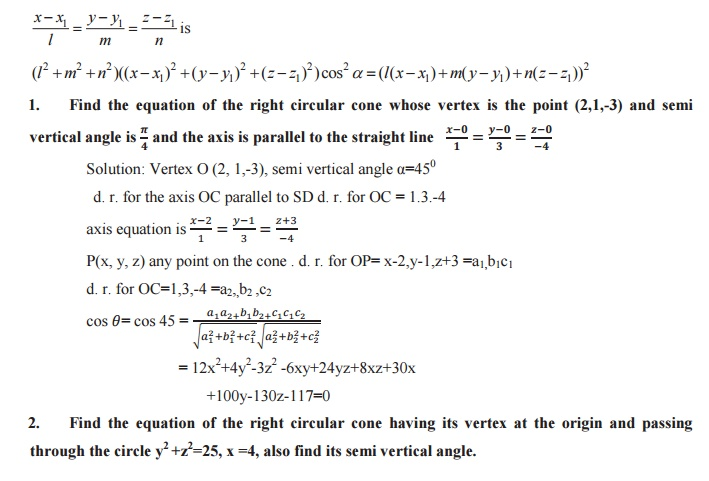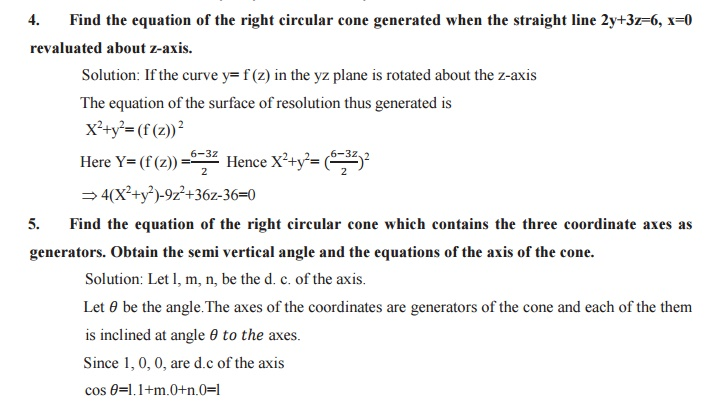CYLINDER

DEFINITION: A cylinder is a surface generated by a straight line which is parallel to a fixed line and it has to intersect a given fixed curve. The straight line is any position called a generator and the fixed point is called the guiding curve of the cylinder.

The equation of cylinders whose generators are parallel to the lineRIGHT CIRCULAR CYLINDER

DEINITION: Right circular cylinder is a surace generated by a straight line which is parallel to a fixed line is at a contant distance it or whose  guiding curve is a circle.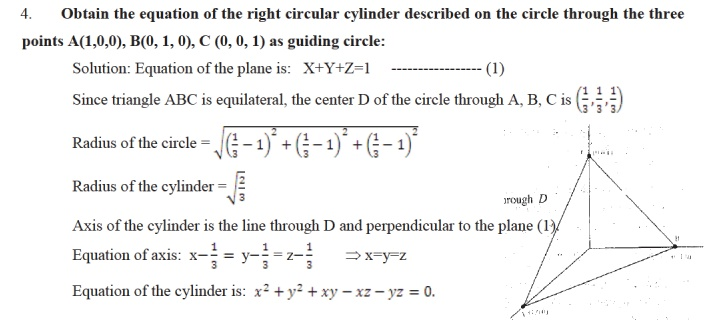THE SPHERE

DEFINITION: A sphere is the locus of a point moving at a constant distance form a fixed point. The  constant distance is the radius and the fixed point is the centre of the sphere.

PLANE SECTION OF A SPHERE:

A plane section of a sphere is a circle sphere S: x2+y2+z2+2ux+2vy+2wz+d=0 plane U: ax+by+cz+d1= 0 the combined equation (S,U) is a circle.

The equation of the sphere through the circle a (S, U ) is S1=S+KU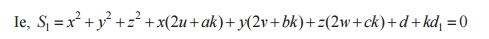EQUATION OF THE TANGENT PLANE

The sphere is x2+y2+z2+2ux+2vy+2wz+d=0  and the point of contact is (x1,y1,z1)  then  Equation of the Tangent plane is xx1+yy1+zz1+ u(x+x1)+v(y+y1)+w(z+z1) +d=0

CONDITION FOR TANGENCY:

Condition for tangency is perpendicular from centre to the plane = radiusCONDITION FOR ORTHOGONALITY OF TWO SPHERES:

The condition for orthogonality of two spheres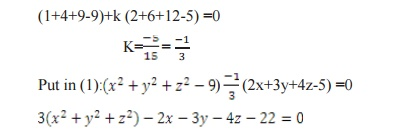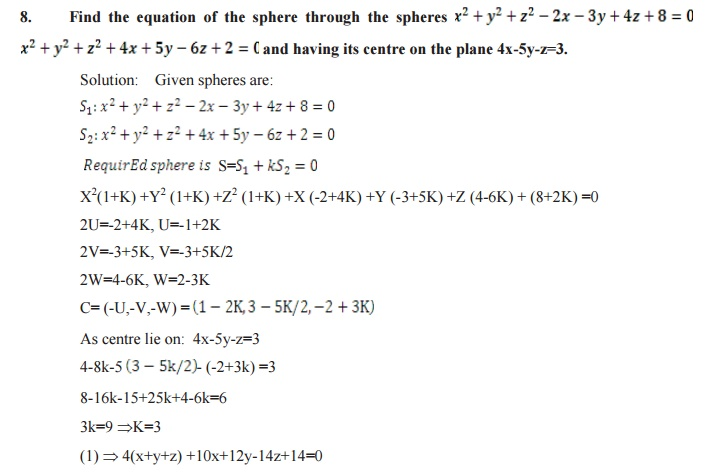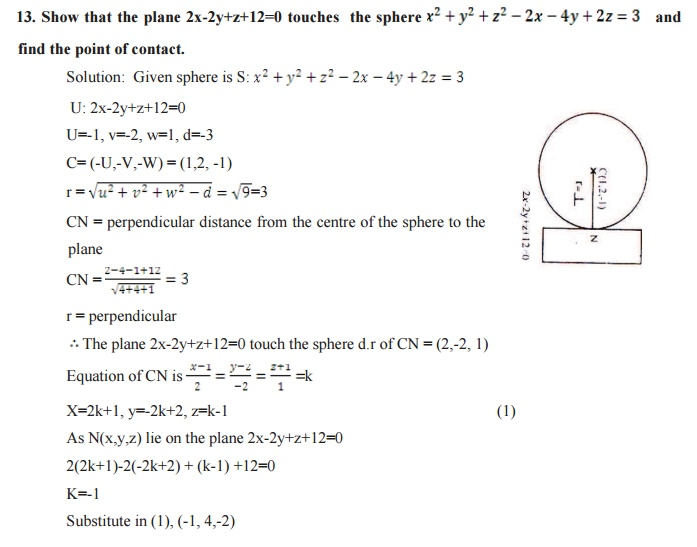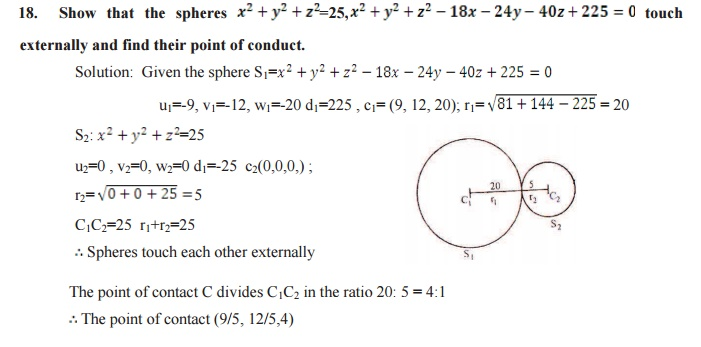Study Material, Lecturing Notes, Assignment, Reference, Wiki description explanation, brief detail
mathematics : Three Dimensional Analytical Geometry : Three Dimensional Analytical Geometry |

Related Topics